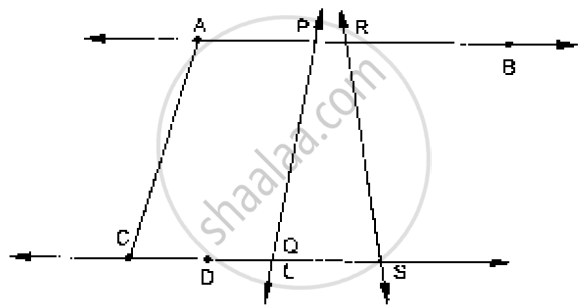Share

Books Shortlist
Your shortlist is empty

# RD Sharma solutions for Class 9 Mathematics chapter 7 - Introduction to Euclid’s Geometry

## Chapter 7 : Introduction to Euclid’s Geometry

#### Page 0

Define the following terms:

Line segment

Define the following term :

Collinear points

Define the following terms :

Parallel lines

Define the following term:

Intersecting lines

Define the following term

Concurrent lines

Define the following term

Ray

Define the following term :

Half-line

How many lines can pass through a given point?

In how many points can two distinct lines at the most intersect?

Given two points P and Q, find how many line segments do they deter-mine.

Name the line segments determined by the three collinear points P, Q and R.

Write the truth value (T/F) of each of the following statements:

Two lines intersect in a point.

Write the truth value (T/F) of each of the following statements:

Two lines may intersect in two points

Write the truth value (T/F) of each of the following statements

A segment has no length.

Write the truth value (T/F) of each of the following statements:

Two distinct points always determine a line.

Write the truth value (T/F) of each of the following statements

Every ray has a finite length.

Write the truth value (T/F) of each of the following statements:

A ray has one end-point only.

Write the truth value (T/F) of each of the following statement:

A segment has one end-point only.

Write the truth value (T/F) of each of the following statements

The ray AB is same as ray BA.

Write the truth value (T/F) of each of the following statement:

Only a single line may pass through a given point.

Write the truth value (T/F) of each of the following statements:

Two lines are coincident if they have only one point in common.

In the below fig., name the following:Fill in the blank so as to make the following statement true:

Two distinct points in a plane determine a ________ line.

Fill in the blank so as to make the following statement true:

Two distinct ________ in a plane cannot have more than one point in common.

Fill in the blank so as to make the following statement

Geven a line and a point, not on the line, there is one and only         line pwahsiscehs through the given point and is       to the given line.

Fill in the blank so as to make the following statement

A line separates a plane into                              parts namely the and the                  itself.

## RD Sharma solutions for Class 9 Mathematics chapter 7 - Introduction to Euclid’s Geometry

RD Sharma solutions for Class 9 Maths chapter 7 (Introduction to Euclid’s Geometry) include all questions with solution and detail explanation. This will clear students doubts about any question and improve application skills while preparing for board exams. The detailed, step-by-step solutions will help you understand the concepts better and clear your confusions, if any. Shaalaa.com has the CBSE Mathematics for Class 9 by R D Sharma (2018-19 Session) solutions in a manner that help students grasp basic concepts better and faster.

Further, we at shaalaa.com are providing such solutions so that students can prepare for written exams. RD Sharma textbook solutions can be a core help for self-study and acts as a perfect self-help guidance for students.

Concepts covered in Class 9 Mathematics chapter 7 Introduction to Euclid’s Geometry are Equivalent Versions of Euclid’S Fifth Postulate, Euclid’S Definitions, Axioms and Postulates, Concept for Euclid’S Geometry.

Using RD Sharma Class 9 solutions Introduction to Euclid’s Geometry exercise by students are an easy way to prepare for the exams, as they involve solutions arranged chapter-wise also page wise. The questions involved in RD Sharma Solutions are important questions that can be asked in the final exam. Maximum students of CBSE Class 9 prefer RD Sharma Textbook Solutions to score more in exam.

Get the free view of chapter 7 Introduction to Euclid’s Geometry Class 9 extra questions for Maths and can use shaalaa.com to keep it handy for your exam preparation

S